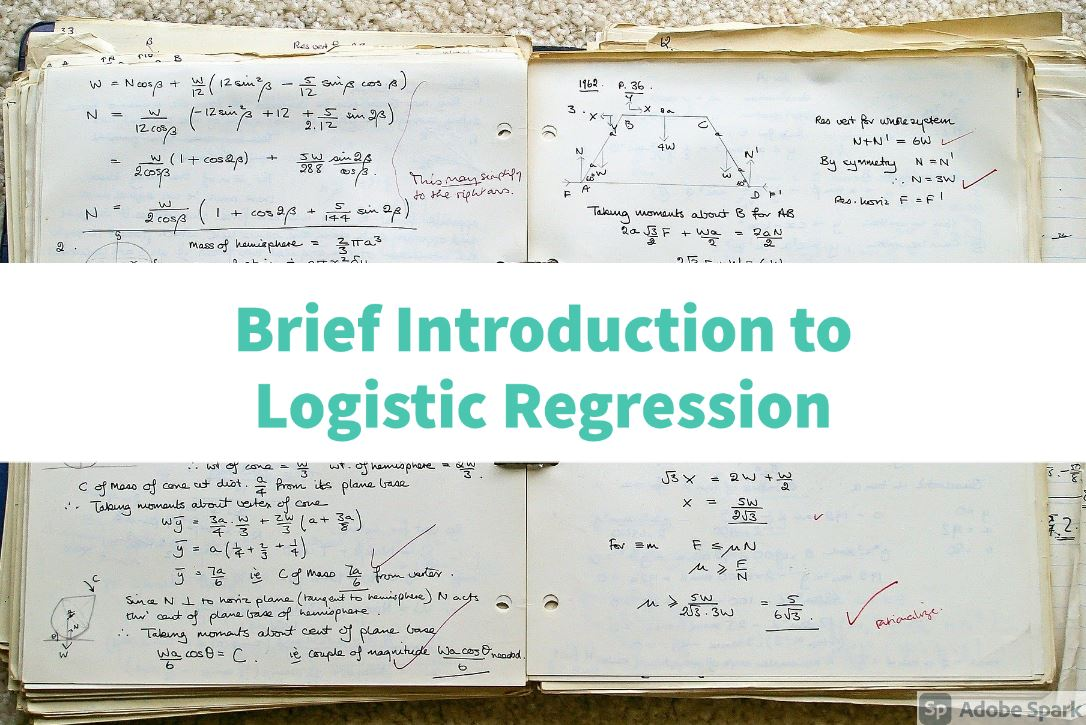Hello every body welcome to another python tutorial, in this tutorial lets see what are variables in python

so basic way of creating a variable in python is first declare name of variable and then assign value in it.

There are some rules while naming variable that are following

A variable can have a short name (like x and y) or a more descriptive name (name, object1 , total_volume).

Rules for Python variables:

• A variable name must start with a letter or the underscore character
• A variable name can only contain alpha-numeric characters and underscores (A-z, 0-9, and _ )
• Variable names are case-sensitive (age, Age and AGE are three different variables)

``````

#Legal variable names:
myvariable = "usman"
my_variable = "usman"
_my_variable = "usman"
myVariable = "usman"
MYVARIABLE = "usman"
myvariable2 = "usman"

#Illegal variable names:
2myvariable = "usman"
my-variable = "usman"
my variable = "usman"

```
```

## Types of variables

There are many types of variables

• int : Integer values or whole number
• float : Decimal values
• str : any thing inside "" or ' '
• boolen : either True or False

Now using variables, Lets create simple basic python program

Following program will add two variable values a and b.

``````
a = 13
b = 2
result = a+b
```
```

Following program will subtract two variable values a and b.

``````
a = 13
b = 2
result = a-b
```
```

Following program will multiply two variable values a and b.

``````
a = 13
b = 2
result = a*b
```
```

Following program will divide two variable values a and b.

``````
a = 13
b = 2
result = a/b
```
```

Following program will divide two variable values a and b ignoring decimal part.

``````
a = 13
b = 2
result = a//b
```
```

### Complete Series